# 天行健 君子当自强而不息

## 3D中的方位和角位移（5）

HTML clipboard HTML clipboard

α = θ/2

|| n || = 1

q = [cosα   n sinα] = [cosα   xsinα   ysinα   zsinα]

q 的对数定义为公式10.15：

log q = log([cosα   n sinα]) ≡ [0  α n ]

≡表示"恒等于"，注意log q 的结果，它一般不是单位四元数。

p = [0  α n ] = [0  (αx   αy   αz)]

|| n || = 1

exp p = exp([0 α n ]) = [cosα  n sinα]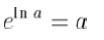exp(log q ) = q

k q = k[w  v ] = [kw   k v ] = k[w  (x  y  z)] = [kw  kx  ky  kz]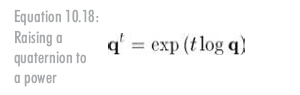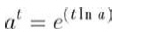Listing 10.1: Code to raise a quaternion to a power

// Quaternion (input and output)
float w,x,y,z;

// Input exponent
float exponent;

// Check for the case of an identity quaternion.
// This will protect against divide by zero
if (fabs(w) < .9999f)
{
// Extract the half angle alpha (alpha = theta/2)
float alpha = acos(w);

// Compute new alpha value
float newAlpha = alpha * exponent;

// Compute new w value
w = cos(newAlpha);

// Compute new xyz values
float mult = sin(newAlpha) / sin(alpha);

x *= mult;
y *= mult;
z *= mult;
}

（1）有必要做单位四元数的检查。因为w=+(-)1会导致mult的计算中出现除零现象。单位四元数的任意次方还是单位四元数。因此，如果检测到输入是单位四元数，忽略指数直接返回原四元数即可。

（2）计算alpha时，使用了acos函数，它的返回值是正的角度。这并不会违背一般性，任何四元数都能解释成有正方向的旋转角度，因为绕某轴的负旋转等价于绕指向相反方向的轴的正旋转。

slerp是一种三元运算，这意味着它有三个操作数。前两个操作数是两个四元数，将在它们中间插值。设这两个"开始"和"结束"四元数分别为 q 0 q 1。插值参数设为变量t，t在0到1之间变化，slerp函数：slerp( q 0, q 1, t)，将返回 q 0 q 1之间的插值方位。

Δa = a1 - a0

lerp(a0, a1, t) = a0 + tΔa

（1）计算两个值的差。

（2）取得差的一部分。

（3）在初始值上加上差的一部分。

（1）计算两个值的差， q 0 q 1的角位移由Δ q = q 0-1 q 1给出。

（2）计算差的一部分，四元数求幂可以做到，差的一部分由(Δ q )t给出。

（3）在开始值上加上差的一部分，方法是用四元数乘法来组合角位移： q 0 q )t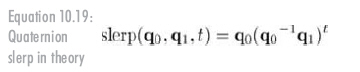slerp的基本思想是沿着4D球面上连接两个四元数的弧插值(这就是球面线性插值这个名称的由来)。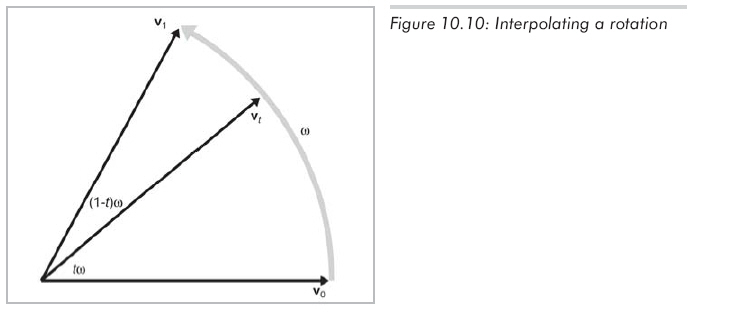v t表达成 v 0 v 1的线性组合，从另一方面说，存在两个非零常数k0和k1，使得：

v t = k0 v 0 + k1 v 1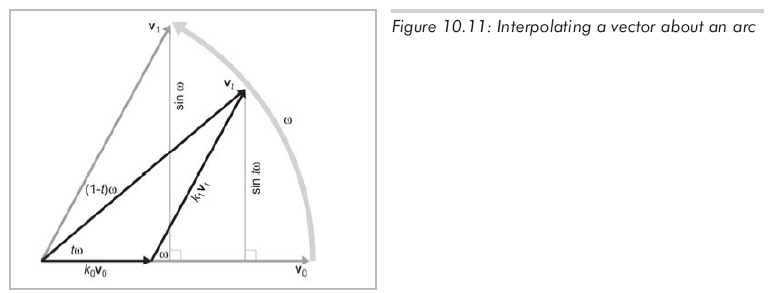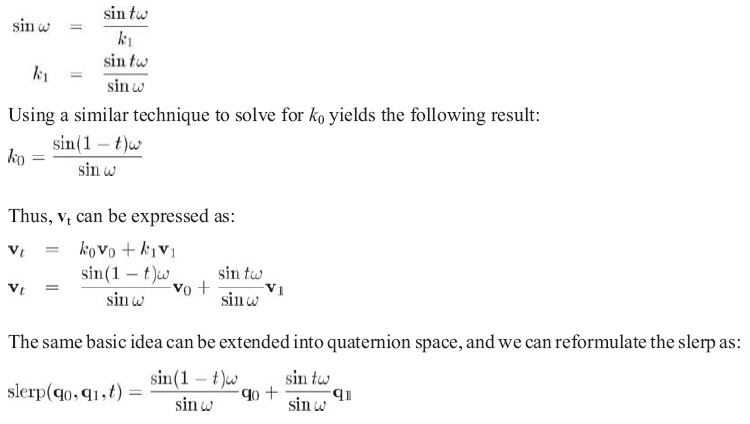Listing 10.2: How slerp is computed in practice

// The two input quaternions
float w0,x0,y0,z0;
float w1,x1,y1,z1;

// The interpolation parameter
float t;

// The output quaternion will be computed here
float w,x,y,z;

// Compute the "cosine of the angle" between the
// quaternions, using the dot product
float cosOmega = w0*w1 + x0*x1 + y0*y1 + z0*z1;

// If negative dot, negate one of the input
// quaternions to take the shorter 4D "arc"
if (cosOmega < 0.0f) {
w1 = –w1;
x1 = –x1;
y1 = –y1;
z1 = –z1;
cosOmega = –cosOmega;
}

// Check if they are very close together to protect
// against divide-by-zero
float k0, k1;

if (cosOmega > 0.9999f) {
// Very close - just use linear interpolation
k0 = 1.0f–t;
k1 = t;
} else {
// Compute the sin of the angle using the
// trig identity sin^2(omega) + cos^2(omega) = 1
float sinOmega = sqrt(1.0f – cosOmega*cosOmega);

// Compute the angle from its sin and cosine
float omega = atan2(sinOmega, cosOmega);

// Compute inverse of denominator, so we only have
// to divide once
float oneOverSinOmega = 1.0f / sinOmega;

// Compute interpolation parameters

k0 = sin((1.0f – t) * omega) * oneOverSinOmega;
k1 = sin(t * omega) * oneOverSinOmega;
}

// Interpolate
w = w0*k0 + w1*k1;
x = x0*k0 + x1*k1;
y = y0*k0 + y1*k1;
z = z0*k0 + z1*k1;

posted on 2008-02-14 12:48 lovedday 阅读(3337) 评论(9)  编辑 收藏 引用### 评论

#### #re: 3D中的方位和角位移（5） 2008-02-27 07:50 梁全

Email：liangquan6@126.com  回复  更多评论

#### #re: 3D中的方位和角位移（5） 2008-02-27 08:48 lovedday

《3D数学基础：图形与游戏开发》  回复  更多评论

#### #re: 3D中的方位和角位移（5） 2008-02-28 08:13 梁全

“继续上面的例子，q4不是预期的绕x轴顺时针旋转240度，而是逆时针80度。”

“继续上面的例子，q4不是预期的绕x轴顺时针旋转120度，而是逆时针？？度。”  回复  更多评论

#### #re: 3D中的方位和角位移（5） 2008-03-05 09:13 梁全

Email:liangquan6@126.com  回复  更多评论

回复  更多评论

#### #re: 3D中的方位和角位移（5） 2014-04-27 11:34 alm

Listing 10.1: Code to raise a quaternion to a power

### 公告• 随笔 - 1360
• 文章 - 75
• 评论 - 520
• 引用 - 0

•